## Transient I and E in LCR Circuits (cont.)

 Voltage is Applied to a De-energized Inductive Circuit An Energized Inductive Circuit is Short Circuited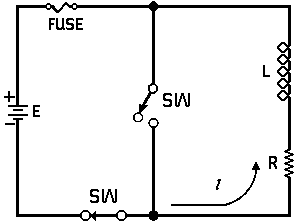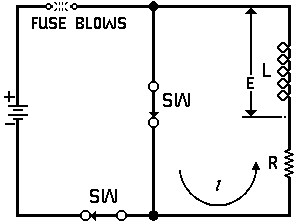E  =  applied potential. E  =  counter potential induced in coil whenSw is closed.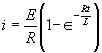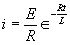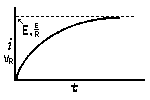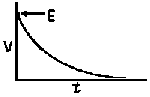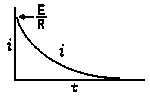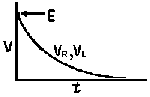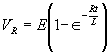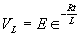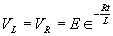In a Capacitive Circuit In an Inductive Circuit In a capacitive circuit, where resistance loss components may be considered as negligible, the flow of current at a given alternating potential of constant frequency, is expressed by In an Inductive circuit, where inherent resistance and capacitance components may be so low as to be negligible, the flow of current at a given alternating potential of a constant frequency, is expressed by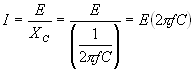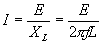where I XC E = = = current in amperes, capacitive reactance of the circuit in ohms, applied potential in volts. where I XL E = = = current in amperes, Inductive reactance of the circuit in ohms, applied potential in volts.